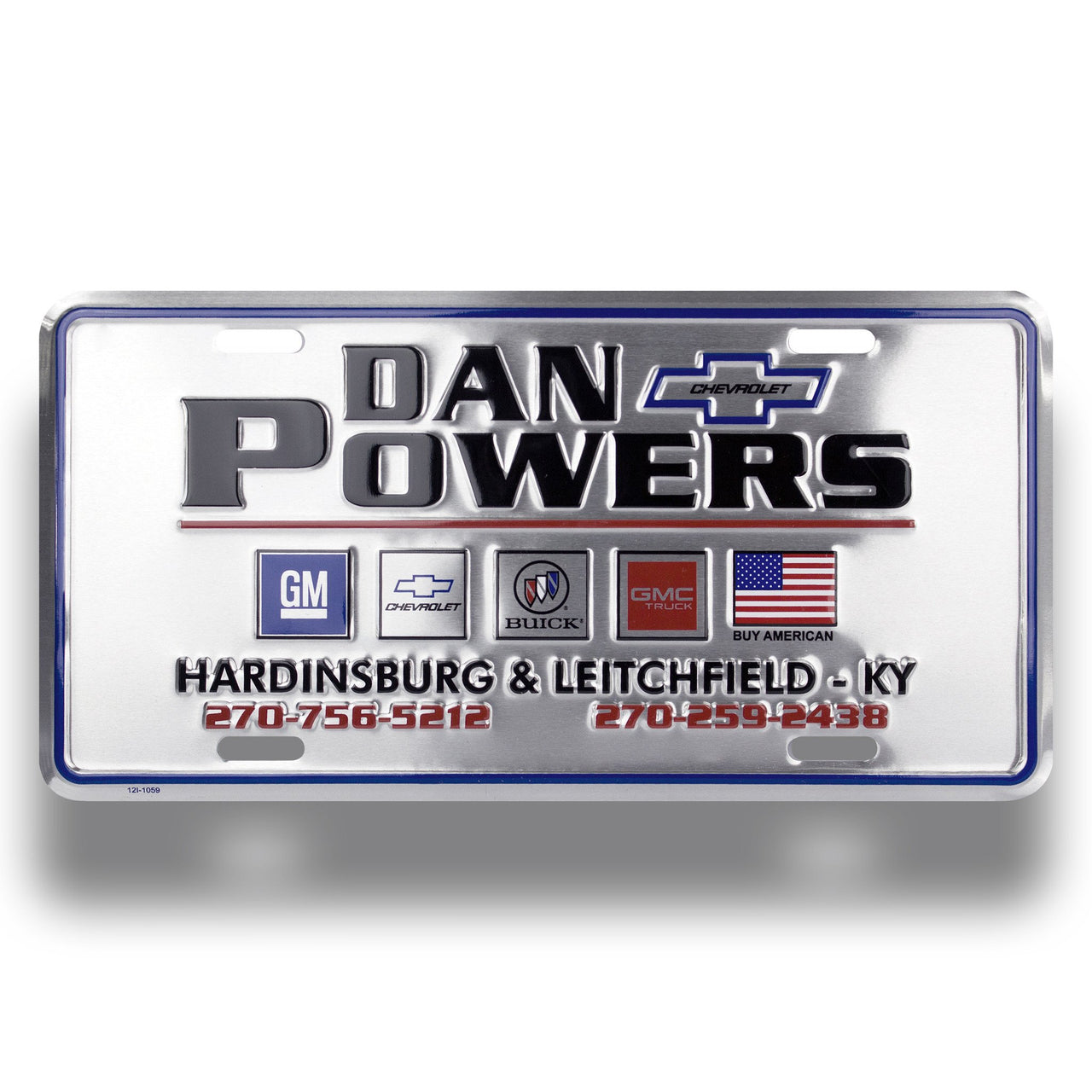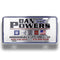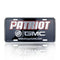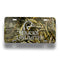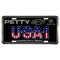• Made of sturdy .024 gauge aluminum
• Each plate is embossed to give the look of raised paint
• Standard size is 12" x 6"
• Price includes one color imprint on white or natural aluminum and four oval holes.
• When using a standard background color other than white, an additional color charge will apply; call for quote
• Full bleed option available at no charge
• Free diamond embossing

Pricing:

• 250= 2.74 each
• 500= 2.08 each
• 1000= 1.79 each
• 2500= 1.69 each
• 5000= 1.59 each

Full Color:

• 250= 3.60 each
• 500= 2.77 each
• 1000= 2.46 each
• 2500= 2.32 each
• 5000= 2.22 each

• 250= .35 each
• 500= .35 each
• 1000= .32 each
• 2500= .29 each
• 5000= .26 each

*\$75 Setup charge

*4-6 week production time

*All orders subject to +/- 5% overrun charge.